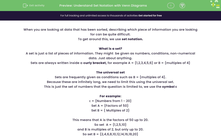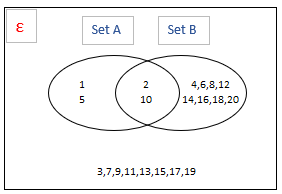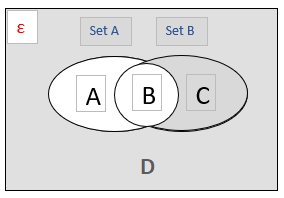# Understand Set Notation with Venn Diagrams

In this worksheet, students will practise using simple set notation and apply it to Venn diagrams.Key stage:  KS 4

Year:  GCSE

GCSE Subjects:   Maths

GCSE Boards:   AQA, Eduqas, Pearson Edexcel, OCR,

Curriculum topic:   Probability

Curriculum subtopic:   Probability Combined Events and Probability Diagrams

Difficulty level:#### Worksheet Overview

When you are looking at data that has been sorted, describing which piece of information you are looking for can be quite difficult.

To get around this, we use set notation.

What is a set?

A set is just a list of pieces of information. They might  be given as numbers, conditions, non-numerical data. Just about anything.

Sets are always written inside a curly bracket, for example A = {1,2,3,4,5,6} or B = {multiples of 4}

The universal set

Sets are frequently given as conditions such as B = {multiples of 4}.

Because these are infinitely long, we need to limit this using the universal set.

This is just the set of numbers that the question is limited to, we use the symbol ε

For example:

ε = {Numbers from 1 - 20}

Set A = {Factors of 50}

Set B = { Multiples of 2}

This means that A is the factors of 50 up to 20.

So set  A = {1,2,5,10}

and B is multiples of 2, but only up to 20.

So set B = {2,4,6,8,10,12,14,16,18,20}

Putting this info into a Venn diagram

All Venn diagrams look something like this:All we have to be able to do is find out which numbers go in which sections.

It's easiest to find B first, as these are the numbers that are in both sets.

B = 2, 10

A is the ones in set A that aren't already in the middle:

A = 1,5

C is the numbers that are in set B that aren't already in the middle:

C = 4,6,8,12,14,16,18,20

D is all the numbers in the universal set that we haven't yet seen:

D = 3,7,9,11,13,15,17,19

This should give the Venn diagram:What other notation do we need?

There are a few other set notations that you need to know for GCSE maths.

Intersection:

This would be written as A∩B

This means all the values that are in A and B.

On the Venn diagram, this is illustrated by:Union:

This would be written as A∪B

This means all the values that are in A or B.

On the Venn diagram, this is illustrated by:Not:

This would be written as A'

This means all the values that are not in A.

On the Venn diagram, this is illustrated by:Example:

ε = {Numbers from 1 - 20}

Set A = { Factors of 50}

Set B = {Multiples of 2}

Find the numbers that satisfy:A∩B

These are the numbers that satisfy both sets A and B and that are less than 21.

The only numbers that are less than 21 as well as being both a factor of 50 and a multiple of 2 are 2 and 10

So, A∩B = 2 and 10

A∪B

This means we want all the numbers that are either a multiple of 2 or a factor of 50 (and still under the limit of 21).

This gives us the numbers 1, 2, 4, 5, 6, 8, 10, 12, 14, 16, 18, 20

A'

Not A means all the numbers under 21 that aren't in set A.

This means the following numbers: 3, 4, 6, 7, 8, 9, 11, 12, 13, 14, 15, 16, 17, 18, 19, 20

Now let's move on to some questions.

### What is EdPlace?

We're your National Curriculum aligned online education content provider helping each child succeed in English, maths and science from year 1 to GCSE. With an EdPlace account you’ll be able to track and measure progress, helping each child achieve their best. We build confidence and attainment by personalising each child’s learning at a level that suits them.

Get started